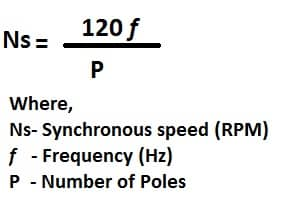# Slip Speed in an Induction Motor

A rotating magnetic field is produced when three phase supply is fed to stator of an induction motor. The rotating magnetic field has constant magnitude and has a circular rotation.The speed at which rotating magnetic field rotates is known as a synchronous speed of the motor. The synchronous speed depends on the number of poles and frequency of the supply source of the motor and it  is denoted by Ns. The synchronous speed (Ns) of the motor is expressed by the following mathematical expression.A  50 Hz and 60 Hz induction motor have following synchronous speed at different number of poles.

The synchronous speed is the speed at which the magnetic field rotates. The synchronous magnetic field gets linked to the rotor conductor and induces voltage in the rotor.The current starts flowing in the rotor because the rotor conductors of the motor is short circuited. The torque is produced in the rotor due to an interaction of magnetic field and the current flowing in the rotor and rotor starts rotating.

Does an induction motor rotates at the synchronous speed? No, if the rotor rotates at the synchronous speed the voltage induced in the rotor would be zero and the rotor current would also be zero.Consequently, the torque of the motor is zero. The motor operation is not possible if the rotor tries to rotate at synchronous speed. The rotor of the induction motor always lags the synchronous speed of the motor.

Definition of Slip

The lagging of the actual speed(N) of the motor with synchronous speed(Ns) is called the slip.The slip of an induction motor is represented by symbol S.The slip of an induction motor is the difference between synchronous speed and actual speed and it  can be mathematically written as ;

S = Ns – N

The actual speed of the motor is always less than the synchronous speed of the motor.

The slip can be represented in the percentage(%) as;

The slip can also be represented in per unit(p.u). The fraction part of the synchronous speed is called the Fractional slip or per unit slip.

The rotor speed of the motor can be given by following mathematical expression.

The slip of an induction motor also can be given in the angular speed of the synchronous speed and the actual speed.

The slip of an induction motor varies from 2 to 4 % .

Illustrative Examples:

A 4 poles, 50 Hz induction motor has slip of 4 %. What is the actual speed of the motor?

The synchronous speed of the 4 Poles,50 Hz induction motor is
Ns= 120 x 50/4 = 1500 RPM.

Slip = 0.04

Actual speed N = Ns(1-S)
N  = 1500(1-0.04)
N  = 1500 x 0.96
N  = 1440 RPM

A 4 poles, 50 Hz induction motor rotor speed is 1480 RPM. What is the slip speed of the motor? The motor draws 500 kW from the supply source. What is the copper loss of the motor.

The synchronous speed of the 4 Poles,50 Hz induction motor is
Ns= 120 x 50/4 = 1500 RPM.

Actual speed (N) = 1480 RPM

Slip(S) = (Ns-N)/Ns x 100

S = (1500-1480)/1500 x 100
S = 20/1500 x 100
S = 1.33 %

Input Power = 500 kW
Copper loss = Slip x Input Power
Copper loss = 0.0133 x 500
Copper loss = 6.66 kWSlip- Torque characteristics of Induction Motor

Want To Learn Faster?

Get electrical, electronic, automation, and instrumentation engineering articles delivered to your inbox every week.

### 2 thoughts on “Slip Speed in an Induction Motor”

1.At the beginning of this page, on the first para and second line, I found that the ‘rotating’ word is mentioned repeatedly. Hence suggested to remove one.

•